# Resolution

Jump to: navigation, search

In homological algebra a right resolution of a moduleis a complex (in homological algebra):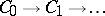, defined for positive degrees and provided with a supplementary homomorphism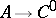such that the sequenceis exact (cf. Exact sequence).

#### Comments

The supplementary homomorphismcan also be seen as a homomorphism of complexes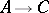, whereis viewed as a complex concentrated in degree zero. The right resolutionis called injective if the modules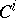are all injective (cf. Injective module). Dually, a left resolution is an exact sequence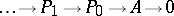. Such a left resolution is called projective if all the modules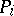are projective, free if all theare free, and flat if all theare flat (cf. Projective module; Flat module).

More generally, the notion of a resolution of an object can be defined in any Abelian category in a completely similar way, [a1]. E.g., in the category of sheaves of Abelian groups on a topological space an injective resolution of a sheafis an exact sequence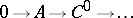of sheaves of Abelian groups with eachan injective sheaf. In sheaf theory one often uses resolutions by flabby or soft sheaves (cf. Flabby sheaf; Soft sheaf). For the case of sheaves over a topos see [a5], [a6].

Resolutions are the main tool in the calculation of derived functors (cf. Derived functor) and in the approach to homology and cohomology as derived functors. In order to construct derived functors in a non-additive category, the technique of simplicial resolutions is used [a4].

In many cases it is useful to use resolutions of very special forms. One such is the resolution afforded by the Koszul complex, which is something like an exterior algebra pulled apart.